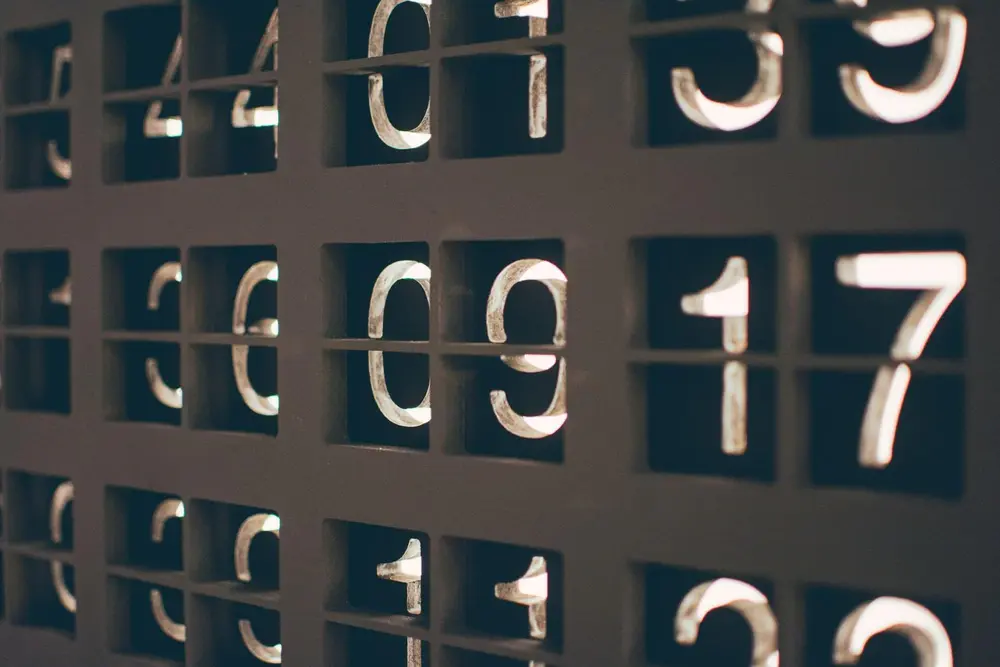# Decimals

Decimals are the part of the number which come after the decimal point. This part of a number shows a part of a whole or 1. You must be comfortable using them as they are used all the time.

Examples:
0.1 is the same as 1/10
0.01 is the same as 1/100
0.001 is the same as 1/1000
0.243 is the same as 234/1000
0.002 is the same as 2/1000
2.0 is the same as 2/1

Terminating Decimals
These are decimals which end straight away (1.5, 0.1234, 1.2345).

Non-Terminating Decimals
These are numbers which have decimals which do not stop. These include numbers such as PI or recurring decimals like a third.

Decimal Places
Decimals may not be very short. Many answers may give a long string of decimal places which don't seem to end. Questions in an exam may ask you to give answers to a specific degree of accuracy.
For example:
If x = 12.3456789
x to 4 significant figures is 12.35
x to 4 decimal places is 12.3457

Number

Number

Number

Number

Number

Number